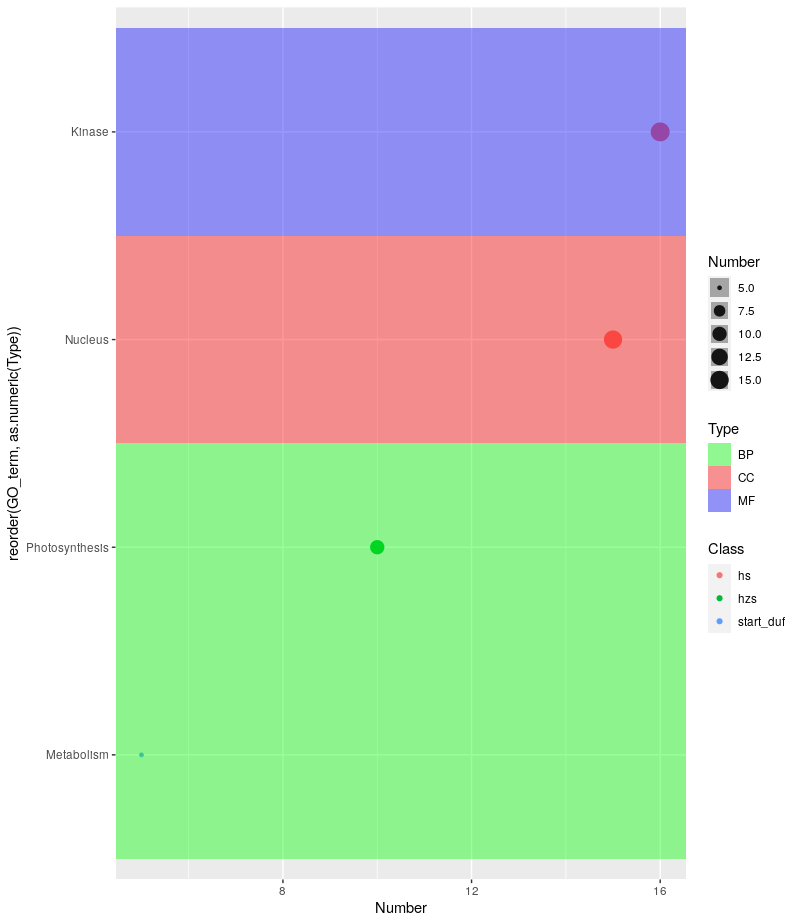# Gene Ontology Bubble Plot using ggplot2

So with your data that seems to look something like this:

``````structure(list(GO_term = structure(c(2L, 4L, 3L, 1L), .Label = c("Kinase",
"Metabolism", "Nucleus", "Photosynthesis"), class = "factor"),
Number = c(5L, 10L, 15L, 16L), Class = structure(c(3L, 2L,
1L, 1L), .Label = c("hs", "hzs", "start_duf"), class = "factor"),
Type = structure(c(1L, 1L, 2L, 3L), .Label = c("BP", "CC",
"MF"), class = "factor")), class = "data.frame", row.names = c(NA,
-4L))
``````

You could work with `geom_tile` and set its `width = Inf` to get something akin to that plot you’re trying to emulate. The use of `forcats::reorder()` within `aes()` is important in order to group the Y-axis values together on the basis of your `Type` column.

``````library(ggplot2)
library(forcats)

ggplot(mydat, aes(y = reorder(GO_term, as.numeric(Type)), x = Number, size = Number)) + geom_point(aes(color = Class), alpha = 1.0) +
geom_tile(aes(width = Inf, fill = Type), alpha = 0.4) +
scale_fill_manual(values = c("green", "red", "blue"))
``````

Which yields:The problem you’d probably run into is having to pass the appropriate number of colors to `scale_fill_manual` for your actual dataset.